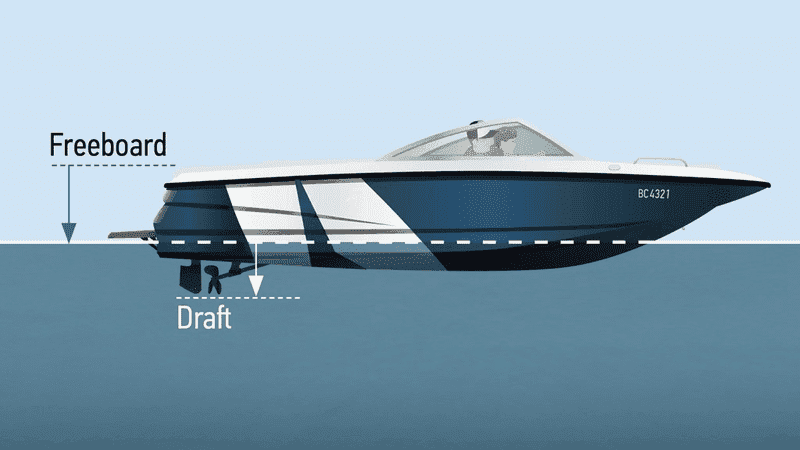# What happens to the buoyant force at the surface of the water?

• I
annamal
TL;DR Summary
What happens to the buoyant force at the surface of the water for an object?
What happens to the buoyant force at the surface of the water for an object? The buoyant force should be greater than the weight of the object if the object were to float up but once the object floats to the surface, there is no more acceleration upwards which means the buoyant force = weight of object, so did the buoyant force decrease in magnitude with less depth in the water? How does that work?

2022 Award
State the magnitude of the buoyant force in general.

State the magnitude of the buoyant force on a sealed bottle of volume ##V## held completely below the surface of water (water's density is ##\rho##).

State the magnitude of the buoyant force on the bottle if it has a fraction ##f## (##0\leq f\leq 1##) of its volume below the water.

An equilibrium is reached when the object floats.
Then the part above the water has mass but lacks the buoyant force of the water.

•russ_watters
annamal
1)State the magnitude of the buoyant force in general.

2) State the magnitude of the buoyant force on a sealed bottle of volume ##V## held completely below the surface of water (water's density is ##\rho##).

3)State the magnitude of the buoyant force on the bottle if it has a fraction ##f## (##0\leq f\leq 1##) of its volume below the water.

1) ##F_b = F_{fl}##
2) ##F_b = \rho Vg##
3) ##F_b = \rho Vf*g##
I see that, but what if the object were completely above the water (no volume below water). According to the equations ##F_b = 0## which doesn't make sense because ##F_b## should equal to weight of object.

Mentor
I see that, but what if the object were completely above the water (no volume below water).
If the object is completely it of the water then something must be holding it up against the force of gravity - maybe it’s hanging from a string? If there’s not something holding it up, it’s going to move downwards under the influence of gravity until the buoyant force becomes equal to its weight and the net force is zero.

•Ibix
annamal
If the object is completely it of the water then something must be holding it up against the force of gravity - maybe it’s hanging from a string? If there’s not something holding it up, it’s going to move downwards under the influence of gravity until the buoyant force becomes equal to its weight and the net force is zero.
No, there is no string hanging from it. So if an object were initially submerged in water, the buoyant force = ##m_{fl}*g## (weight of fluid displaced) and then it increased to ##m_{obj}*g##?

2022 Award
No, there is no string hanging from it.
Then how is it completely out of the water? Even very low density objects floating have some part below the waterline.

I see that, but what if the object were completely above the water (no volume below water).
Then buoyancy would be irrelevant.
The object must be lighter than air, or supported by some other mechanism.

Homework Helper
Possibly worth mentioning is that "buoyancy" is nothing more or less than the effect of higher fluid pressure on the bottom of an object compared to lower fluid pressure on its top.

For a fluid that is in equilibrium, the difference in the top and bottom forces will turn out to be exactly equal to the weight of the fluid that could otherwise occupy the volume of the object.

The idea is if the fluid that is displaced was in equilibrium then the supporting pressure force difference must have been exactly enough to support the fluid's weight. When that fluid is replaced by an object of the same weight, equilibrium is maintained

annamal
Then how is it completely out of the water? Even very low density objects floating have some part below the waterline.
You mean completely on the surface of the water not out of the water. My question is theoretical.

2022 Award
You mean completely on the surface of the water not out of the water. My question is theoretical.
You asked about the case where the object is completely out of the water. For that to happen the object must be suspended somehow, or else it will sink slightly into the water. You have said both that it isn't suspended and that it isn't sinking into the water, which is contradictory.

For an object that is less dense than water the buoyant force is greater than its weight when it is completely submerged, so it will rise unless it is held down. If it is completely out of the water the buoyant force is zero, so the object will fall unless it is suspended. There is an equilibrium point somewhere when the object is partially submerged where the buoyant force equals the weight, and this is where the object floats.

Staff Emeritus
There is no such thing as a boat "on the water". A portion is always below the water. The part below the water moves aside some water to make room. Magically, the weight of the water moved aside equals the weight of the boat. That is called https://en.wikipedia.org/wiki/Archimedes'_principleWhen this ship is on the water, the red part goes under the water.•Ibix